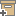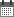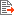ניהול
קהילה:
אסיף מאגר המחקר החקלאי
From four-taxon trees to phylogenies: The case of mammalian evolutionBack to searchPrevious item
Next item
Year:
1998
Authors :
אופיר, רון
;
.
Volume :
Co-Authors:
Ben-Dor, Amir, Dep of Computer Science, Haifa, Israel
Chor, Benny, Dep of Computer Science, Haifa, Israel
Graur, Dan, Dep of Computer Science, Haifa, Israel
Ophir, Ron, Dep of Computer Science, Haifa, Israel
Pelleg, Dan, Dep of Computer Science, Haifa, Israel
Facilitators :
From page:
9
To page:
19
(
Total pages:
11
)
Abstract:
In this work we present two new approaches for constructing phylogenetic trees. The input is a list of weighted quartets over n taxa. Each quartet is a subtree on four taxa, and its weight represents a confidence level for the specific topology. The goal is to construct a binary tree with n leaves such that the total weight of the satisfied quartets is maximized (an NP hard problem). The first approach we present is based on geometric ideas. Using semidefinite programming, we embed the n points on the n-dimensional unit sphere, while maximizing an objective function. This function depends on Euclidean distances between the four points, and reflects the quartet topology. Given the embedding, we construct a binary tree by performing geometric clustering. This process is similar to the traditional neighbor joining, with the difference that the update phase retains geometric meaning: When two neighbors are joined together, their common ancestor is taken to be the center of mass of the original points. The geometric algorithm runs in poly(n) time, but there are no guarantees on the quality of its output. In contrast, our second algorithm is based on dynamic programming, and it is guaranteed to find the optimal tree (with respect to the given quartets). Its running time is a modest exponential, so it can be implemented for modest values of n. We have implemented both algorithms, and ran them on real data for n = 15 taxa (14 mammalian orders and an outgroup taxon). The two resulting trees improve previously published trees and seem to be of biological relevance. Interestingly, the geometric algorithm produced a tree whose score is 98.2% of the optimal value on this input set (72.1% vs. 73.4%). This gives rise to the hope that the geometric approach will prove viable even for larger cases where the exponential, dynamic programming approach is no longer feasible.
Note:
Related Files :
Algorithms
biology
Computational complexity
Dynamic programming
Geometric clustering
Phylogenetic tree
Topology
Trees (mathematics)
עוד תגיות
תוכן קשור
More details
DOI :
Article number:
Affiliations:
Database:
סקופוס
Publication Type:
מאמר מתוך כינוס
;
.
Language:
אנגלית
Editors' remarks:
ID:
31753
Last updated date:
02/03/2022 17:27
Creation date:
17/04/2018 01:05Scientific Publication
From four-taxon trees to phylogenies: The case of mammalian evolution
Ben-Dor, Amir, Dep of Computer Science, Haifa, Israel
Chor, Benny, Dep of Computer Science, Haifa, Israel
Graur, Dan, Dep of Computer Science, Haifa, Israel
Ophir, Ron, Dep of Computer Science, Haifa, Israel
Pelleg, Dan, Dep of Computer Science, Haifa, Israel
From four-taxon trees to phylogenies: The case of mammalian evolution
In this work we present two new approaches for constructing phylogenetic trees. The input is a list of weighted quartets over n taxa. Each quartet is a subtree on four taxa, and its weight represents a confidence level for the specific topology. The goal is to construct a binary tree with n leaves such that the total weight of the satisfied quartets is maximized (an NP hard problem). The first approach we present is based on geometric ideas. Using semidefinite programming, we embed the n points on the n-dimensional unit sphere, while maximizing an objective function. This function depends on Euclidean distances between the four points, and reflects the quartet topology. Given the embedding, we construct a binary tree by performing geometric clustering. This process is similar to the traditional neighbor joining, with the difference that the update phase retains geometric meaning: When two neighbors are joined together, their common ancestor is taken to be the center of mass of the original points. The geometric algorithm runs in poly(n) time, but there are no guarantees on the quality of its output. In contrast, our second algorithm is based on dynamic programming, and it is guaranteed to find the optimal tree (with respect to the given quartets). Its running time is a modest exponential, so it can be implemented for modest values of n. We have implemented both algorithms, and ran them on real data for n = 15 taxa (14 mammalian orders and an outgroup taxon). The two resulting trees improve previously published trees and seem to be of biological relevance. Interestingly, the geometric algorithm produced a tree whose score is 98.2% of the optimal value on this input set (72.1% vs. 73.4%). This gives rise to the hope that the geometric approach will prove viable even for larger cases where the exponential, dynamic programming approach is no longer feasible.
Scientific Publication
You may also be interested in